# Solution assignment 22 Product and Quotient rule

### Assignment 22

Differentiate: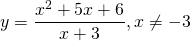### Solution

This function can be differentiated by applying the quotient rule. This method is straightforward.
With functions like this it is advisable to verify whether either the numerator or the denominator can be factorized. This often results in a function which can be differentiated easily.
In this case we can rewrite the numerator: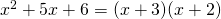And then the function becomes for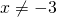: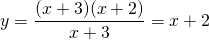which has a derivative.

Note that both the original function and the derivative are discontinuous for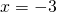and thus cannot be differentiated.

0